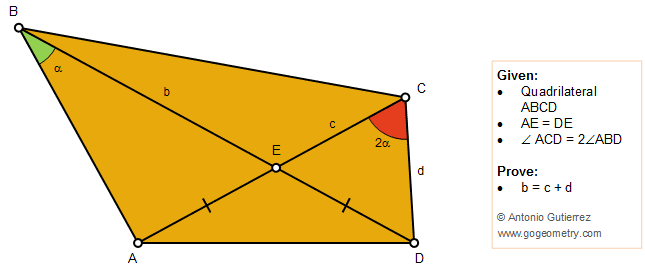# Geometry Problem 1387: Quadrilateral, Double Angle, Congruence, Isosceles Triangle

### Proposition

The figure below shows a quadrilateral ABCD with diagonals AC and BD intersecting at E so that AE = DE and the angle ACD is double the angle ABD. Prove that BE = CE + CD